This is my first blog post on data science prophet.

Office meeting rooms are mostly occupied especially in summer and financial year-end. In This article lets try to dive into it using machine learning that how other factors influence the decision to occupy a meeting room with the help of data.

In this post we are going to classify whether an office room will be occupied or not based on features like temperature, lighting, humidity etc

Dataset we have used it from UCI data repository Dataset

Variable Information

Year- Year

Month – month of the year

weekend- whether it is Saturday or Sunday

day of the week – starting from Monday labelled as 1 to 7

Temperature, in Celsius

Relative Humidity, %

Light, in Lux

CO2, in ppm

Humidity Ratio, Derived quantity from temperature and relative humidity, in kgwater-vapor/kg-air

Occupancy, 0 or 1, 0 for not occupied, 1 for occupied status

This is a binary classification problem

I have made some feature engineering in the original data hence updated data can be found from my (Github Link).
I have done feature engineering on Date columns and extracted useful feature like Year, Month, Weekend, Day of the week etc to provide some more insights

Install the latest Pycaret build (current version 2.2.0)

`!pip install pycaret`

For windows go to cmd and type

`pip install pycaret`

Import useful libraries

``` import pandas as pd import numpy as np from pycaret.classification import *```

Only for google colab – it enables interactive console for google colab

``` from pycaret.utils import enable_colab enable_colab()```

``` df = pd.read_csv("occupancy_office2.csv") df.head() ```Let’s Find some descriptive statistics on our dataset
``` df.describe().T```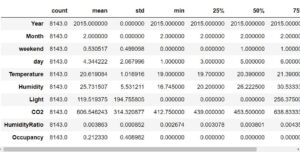We will find the frequency count on the target variable Occupancy, and we can find that there is a class imbalance but thankfully Pycaret takes care of the imbalance class using inbuilt SMOTE technique.
``` df['Occupancy'].value_counts()```

Now lets start building the model. Pycaret makes our life very easy with just one line code to setup the classification model. If you have GPU, you can enable it using “use_gpu = True” during the setup. Here session id is similar to random seed we use, to reproduce the same result every time

# Importing module and initializing setup
``` from pycaret.classification import * clf1 = setup(data = df, target = 'Occupancy',session_id = 1111)```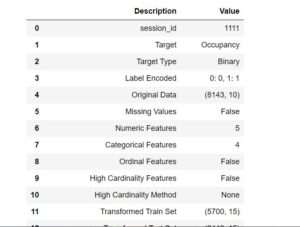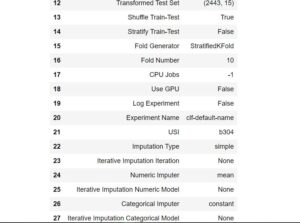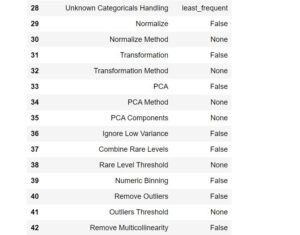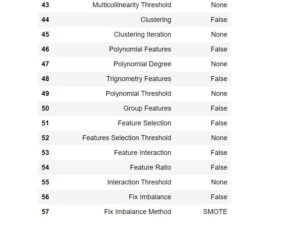Once the Setup is done Pycaret has this awesome functionality which can compare all the available model for the classification task which we have provided. It will create a neat table to let us know which model is performing the best on this data
``` compare_models()```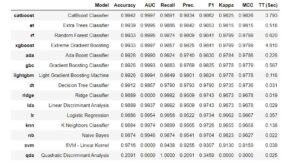From the compare models we can see catboost classifier works the best in our data. hence, lets create our model using catboost classifier. It will create 10 different models on the data and will provide the summary statistics on the model performance
``` catboost_model = create_model('catboost')```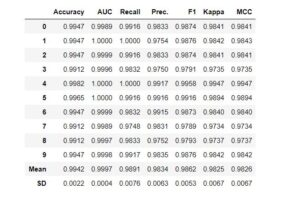Only drawback with pycaret is that current version 2.2 does not have ability to create confusion matrix and other plots for Catboost model.

Hyperparameter tuning

Tuning hyperparameters of a machine learning model in any module is as simple as writing tune_model. . Here our target class is imbalanced hence we will tune F1 as F1 is the harmonic mean of precision and recall and will be the best performance measure for our model

``` tuned_catboost = tune_model(catboost_model,optimize= 'F1')```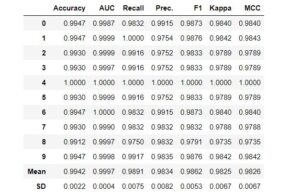after hyperparameter tuning we can see that Average F1 score is coming around 98% which means the model is learning on the training data well and should surely perform well when the new data is provided for the prediction
``` final_stack = finalize_model(tuned_catboost)```
``` save_model(final_stack,'catboost classifier 17dec2020')```

Pycaret has an inbuilt pipeline function which can save all the changes made to the dataset during training period and can apply all the changes when the new data is provided to the model.
We will finalize this model and save it to be used for the future prediction.

``` saved_final_catboost = load_model('catboost classifier 17dec2020')```

Now Lets check the model performance on a random data point in our dataset
``` import random random.seed(101) random_index = random.randint(0,len(df))```
``` new_occupant = pd.DataFrame(df.drop('Occupancy',axis=1).iloc[random_index ]).T new_occupant```#saved_final_catboost.predict(new_occupant)
``` predict_model(saved_final_catboost, data=new_occupant)```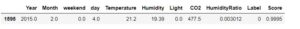``` df.iloc[random_index ]['Occupancy']```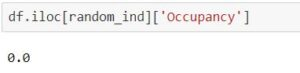As you can see the actual Occupancy was as 0 and predicted class is also 0 with 99% confidence.

In The next tutorial we will be working on the deployment of this model in the form of Rest API using Fastapi. Stay tuned for some more informative articles

Above code and Notebook is available in my Github repo for your reference.

—————- References ————————-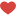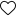## Join the Conversation

1.1 Comment